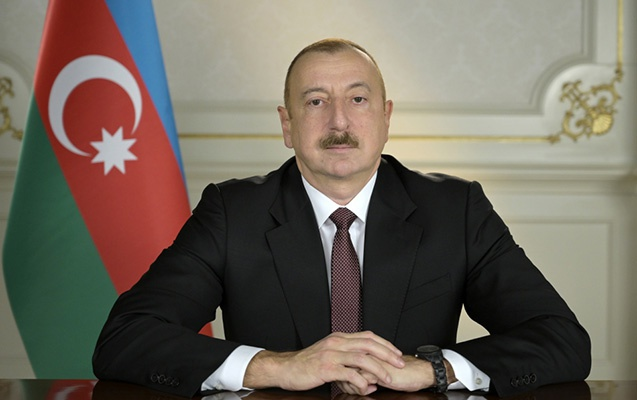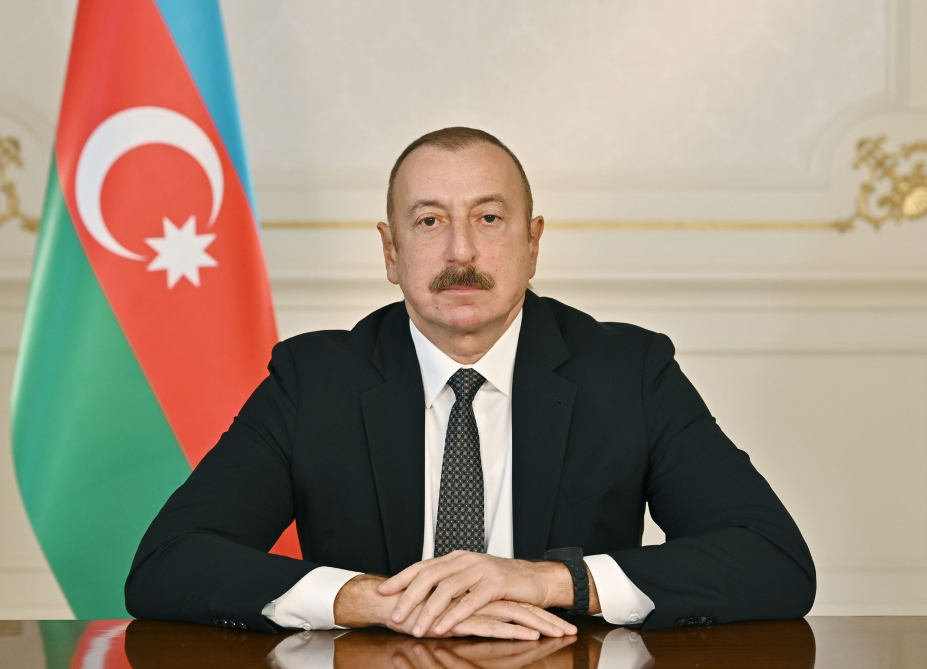Azərbaycan Respublikası Elm və Təhsil Nazirliyi
Riyaziyyat və Mexanika İnstitutu

## Qarabağ xəbərləri### Azərbaycan Respublikasında 2023-cü ilin “Heydər Əliyev İli” elan edilməsi haqqında Azərbaycan Respublikası Prezidentinin Sərəncamı### Azərbaycan Respublikasında 2022-ci ilin “Şuşa İli” elan edilməsi haqqında Azərbaycan Respublikası Prezidentinin Sərəncamı## Transactions 2012/1/XXXII

 Year: 2012 Volume: 1 Number: XXXII Aslanov H.I., Badalova K.H. Abstract PDF On asymptotic distribution of eigenvalues of 2-nd order operator-differential equations on a semi-axis [PDF] 3 Aslanova N.M., Aslanov Kh.M. Abstract [PDF] Asymptotics of eigenvalue distribution and trace formula of one singular [PDF] 9 Babayev A. M-B. Abstract [PDF] On relation between the norm and the quasinorm of the sum of polynomials dependent on di®erent variables [PDF] 25 Dadashova I.B. Abstract [PDF] Analogue of J.Walsh problem in integral metric on curves in a complex plane [PDF] 31 Gasanova A.I. Abstract [PDF] Determination of the coeffcient of parabolic equation in the problem with nonlinear boundary condition [PDF] 39 Gasumova S.H. Abstract [PDF] Two-weighted inequality for parabolic singular integral operators in vector-valued Lebesgue spaces [PDF] 45 Guliyev H.F., Mustafayeva T.M. Abstract [PDF] Optimal stabilization for a weak nonlinear hyperbolic equation with phase restriction [PDF] 53 Guliyev V.S., Shukurov P.S. Abstract [PDF] Adams type result for sublinear operators generated by Riesz potentials on generalized Morrey spaces [PDF] 61 Guseynov R.V., Aliyev N.A., Salmanova Sh.Yu. Abstract [PDF] First order partial equations having a unique solution [PDF] 71 Iskenderov B.A., Akhundova G.Kh., Suleymanov S.E. Abstract [PDF] Asymptotic expansion of the Cauchy problem solution at large values of time for Barenblatt-Jeltov-Kochina equation [PDF] 77 Jamshidipour A.H., Huseynov H.M. Abstract [PDF] Solution of the inverse scattering problem for the Sturm-Liouville equation with a spectral parameter in discontinuity condition [PDF] 89 Khanmamedov A.Kh., Masmaliyev H.M. Abstract [PDF] A solution method for a system of nonlinear differential equations [PDF] 101 Rzaev R.M., Musayev A.M. Abstract [PDF] On approximation of functions by Mellin singular integrals [PDF] 107 Sabzaliev M.M. Abstract [PDF] On asymptotics of solution of a boundary value problem for a quasilinear elliptic equation degenerating to a parabolic equation [PDF] 117 Tagiyev H.T. Abstract [PDF] On the existence of the solution of nonlocal condition optimal control problem for hyperbolic type equation [PDF] 127
Azərbaycanda COVID-19 ilə bağlı statistika
• Virusa yoluxan

832809

• Sağalan

822263

• Yeni yoluxan

0

• Aktiv xəstə

225

• Ölüm halı

10321

• Test edilib

7,684,217